## How To Use The RANDBETWEEN Function In Google Sheets

The RANDBETWEEN function in Google Sheets is used to generate a random integer (whole number) between two values.

For example, this RANDBETWEEN formula generates a random number between 1 and 10:

`=RANDBETWEEN(1,10)`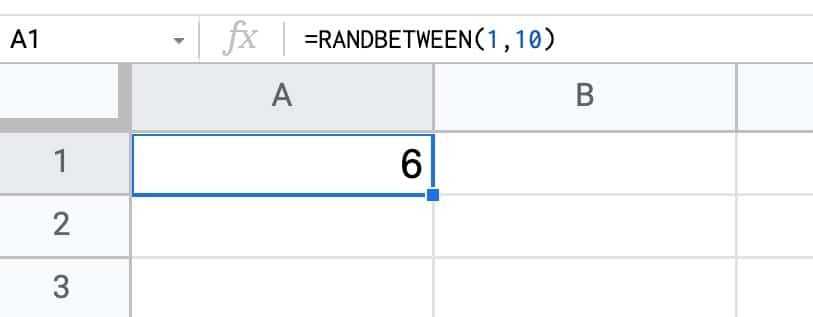The function will generate a new random number each time your Sheet changes.

## How To Create A Random Number Generator In Google Sheets

The RAND function in Google Sheets generates a random number between 0 and 1 and can be used as a random number generator in Google Sheets.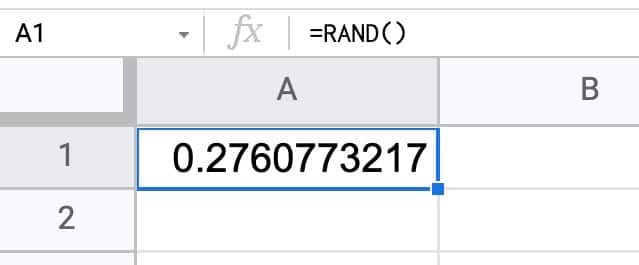The RAND function outputs a decimal number between 0 and 1, for example, 0.2760773217.

## Formula Challenge #6: Split A String Into Characters And Recombine In Random Order

This Formula Challenge originally appeared as Tip #194 of my weekly Google Sheets Tips newsletter, on 7 March 2022.

Congratulations to everyone who took part and well done to the 97 people who submitted a solution!

Special mention to Kieran D., Louise A., Martin H., Jelle G., Karl S., Earl N., Doug S., JP C., Alan B., and others for their ingenious solutions. I’ve shared the best below.

Sign up here so you don’t miss out on future Formula Challenges:

## The Challenge Part I: Split A String Into Characters

Question: can you create a single formula to split a string into characters so that each character is in its own cell?

i.e. can you create a single formula in cell B1 that creates the output shown in this example: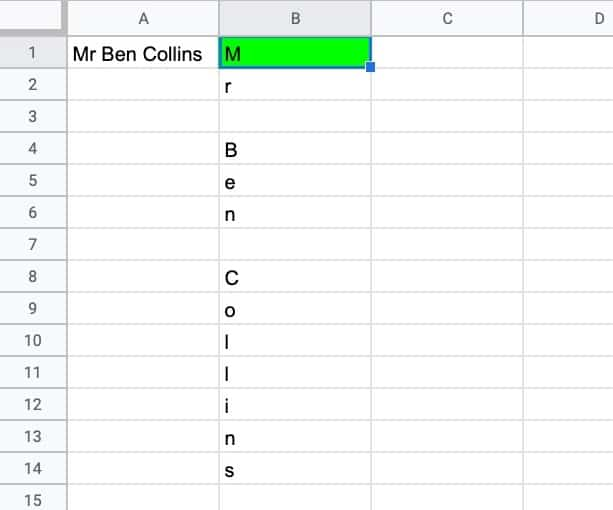## How To Use The RANDARRAY Function In Google Sheets

The RANDARRAY function in Google Sheets generates an array of random numbers between 0 and 1. The size of the array output is determined by the row and column arguments.

Here’s a RANDARRAY formula that generates an array of random numbers between 0 and 1, across 10 rows and 3 columns:

`=RANDARRAY(10, 3)`

which gives an output: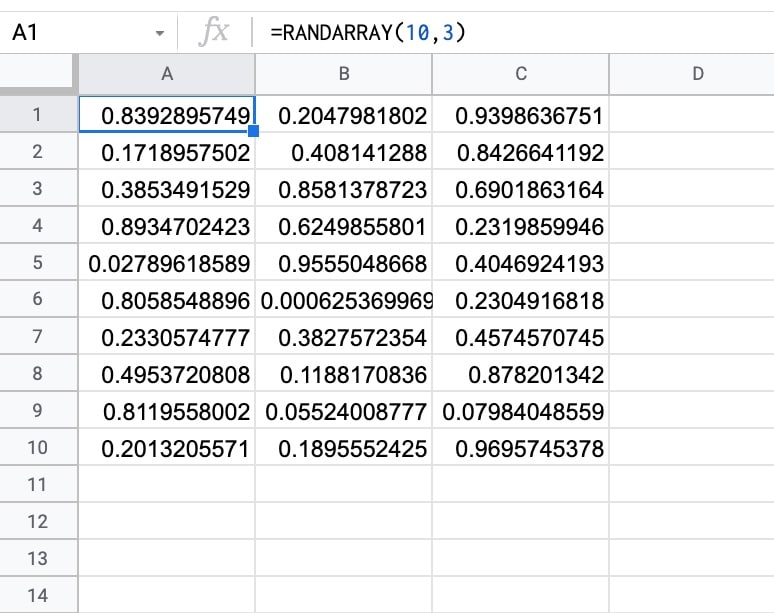🔗 The RANDARRAY template is available at the bottom of this article.

The Google Sheets SUMIFS function is used to sum ranges based on conditional tests. In other words, the SUMIFS in Google Sheets adds values to a total only when multiple conditions are met.

Suppose we want to calculate the total for Large products in New York: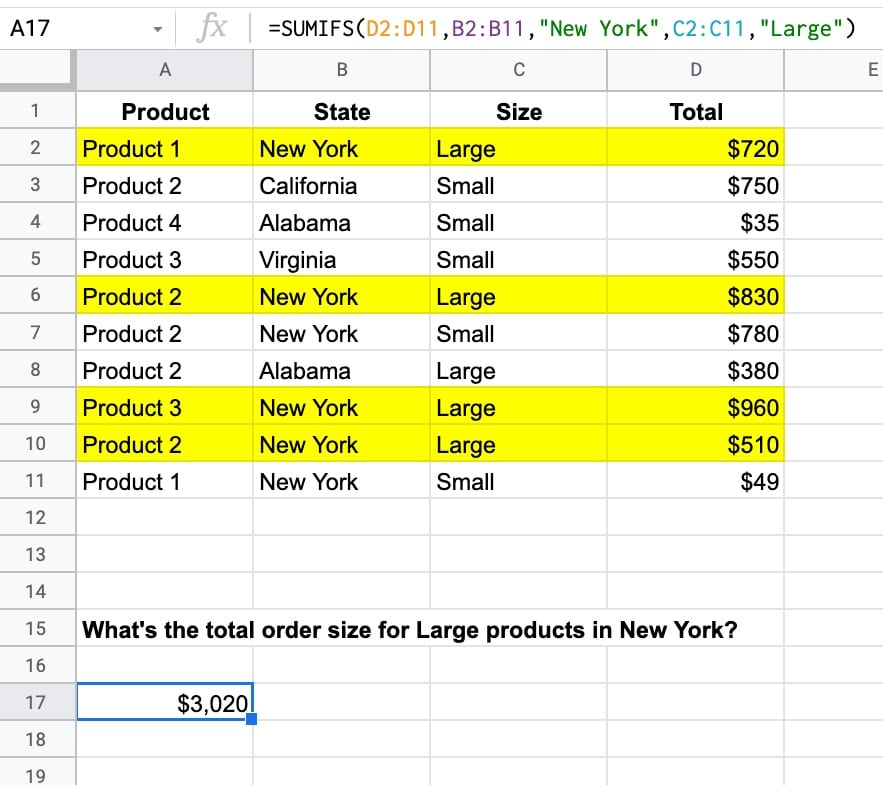The SUMIFS function to calculate the total for two conditions, Large and New York, is:

`=SUMIFS(D2:D11,B2:B11,"New York",C2:C11,"Large")`

which gives an answer of \$3,020.

In this case, there are four rows, highlighted in yellow, that match the criteria of New York in column B and Large in column C.

The total values of these four rows are added together by the SUMIFS function, all the other rows are discarded.

🔗 Get this example and others in the template at the bottom of this article.